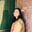Trusted answers to developer questions
Trusted Answers to Developer Questions

Related Tags

numpy
python

# What is numpy.isposinf() in Python?Umme Ammara

Grokking Modern System Design Interview for Engineers & Managers

Ace your System Design Interview and take your career to the next level. Learn to handle the design of applications like Netflix, Quora, Facebook, Uber, and many more in a 45-min interview. Learn the RESHADED framework for architecting web-scale applications by determining requirements, constraints, and assumptions before diving into a step-by-step design process.

Python’s numpy.isposinf() is used to test if an element is positive infinity or not. It tests an array element-wise and returns a boolean array as the output.

## Syntax

numpy.isposinf is declared as follows:

numpy.isposinf(x, out=None)


In the syntax above, x is the non-optional parameter, and out is the optional parameter.

## Parameters

The numpy.isposinf() method takes the following compulsory parameter:

• x [array-like] - input array.

The numpy.isposinf() method takes the following optional parameter:

• out [array_like, optional] - represents the location into which the output of the method is stored. If out is not specified or is None, a freshly-allocated boolean array is returned.

## Return value

numpy.isposinf returns a boolean array with the same dimensions as x. The method returns True /False or 0/1 depending on the following factors:

• If out is not specified, a boolean array is returned. If the element is positive infinity, True is returned. Otherwise, False is returned.

• If out is specified and the type is a numerical array, the result is a numerical array. The integer value 1 is returned if the element is positive infinity and returns 0 otherwise.

An error occurs in the case of the following:

• If x is scalar and out is specified.

• If x and out have different shapes.

• If x contains complex values.

## Examples

The example below shows the use of numpy.isposinf() on the elements a and b:

import numpy as npa = -np.infb = np.infprint (np.isposinf(a))print (np.isposinf(b))

The following example shows the use of numpy.isposinf() on the array arr1 when out is not specified:

import numpy as nparr1 = np.array([2, np.inf, 0, np.nan])print (np.isposinf(arr1))

The following example shows the use of numpy.isposinf() on the array arr2 when out is specified:

import numpy as nparr2 = np.array([np.inf, 2, 0])out = np.array([1,1,1])print (np.isposinf(arr2,out))

RELATED TAGS

numpy
python

CONTRIBUTORUmme Ammara